# An Aperiodic Set of Eleven Wang Tiles

AUTHORS : B. Durand and A. Shen :
EDITOR/ART : R. Ghrist :

This is a review of E. Jeandel and M. Rao, “An aperiodic set of 11 Wang tiles”, Advances in Combinatorics, 2021, https://doi.org/10.19086/aic.18614; see also the preprint https://arxiv.org/abs/1506.06492 from 2015.

HISTORIC ACCOUNT. Assume that a finite set of geometric shapes, called tiles, is given. We want to tile the entire plane without gaps and overlaps by tiles. Is it possible? What is the structure of possible tilings? Under what conditions does a tile set force aperiodic tilings? For a classic example of an aperiodic tiling, see the Penrose tiles in Figures 1 and 2.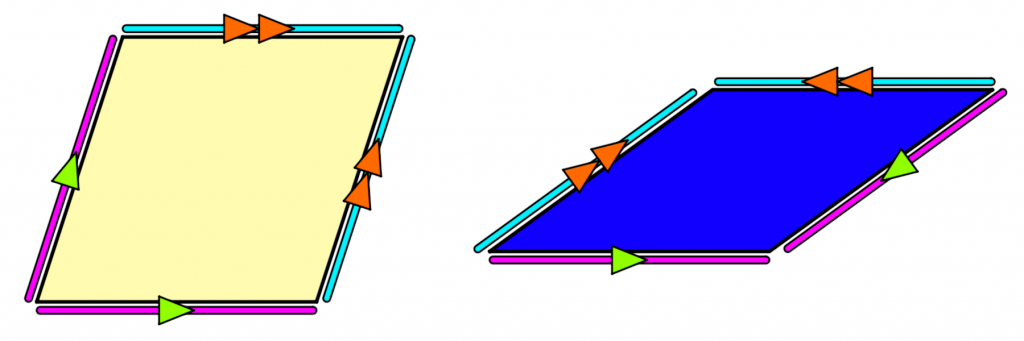Figure 1 : In the classic case of Penrose tiles, two rhombic tiles can make periodic or aperiodic tilings of the plane. One can impose matching rules by decorating the edges.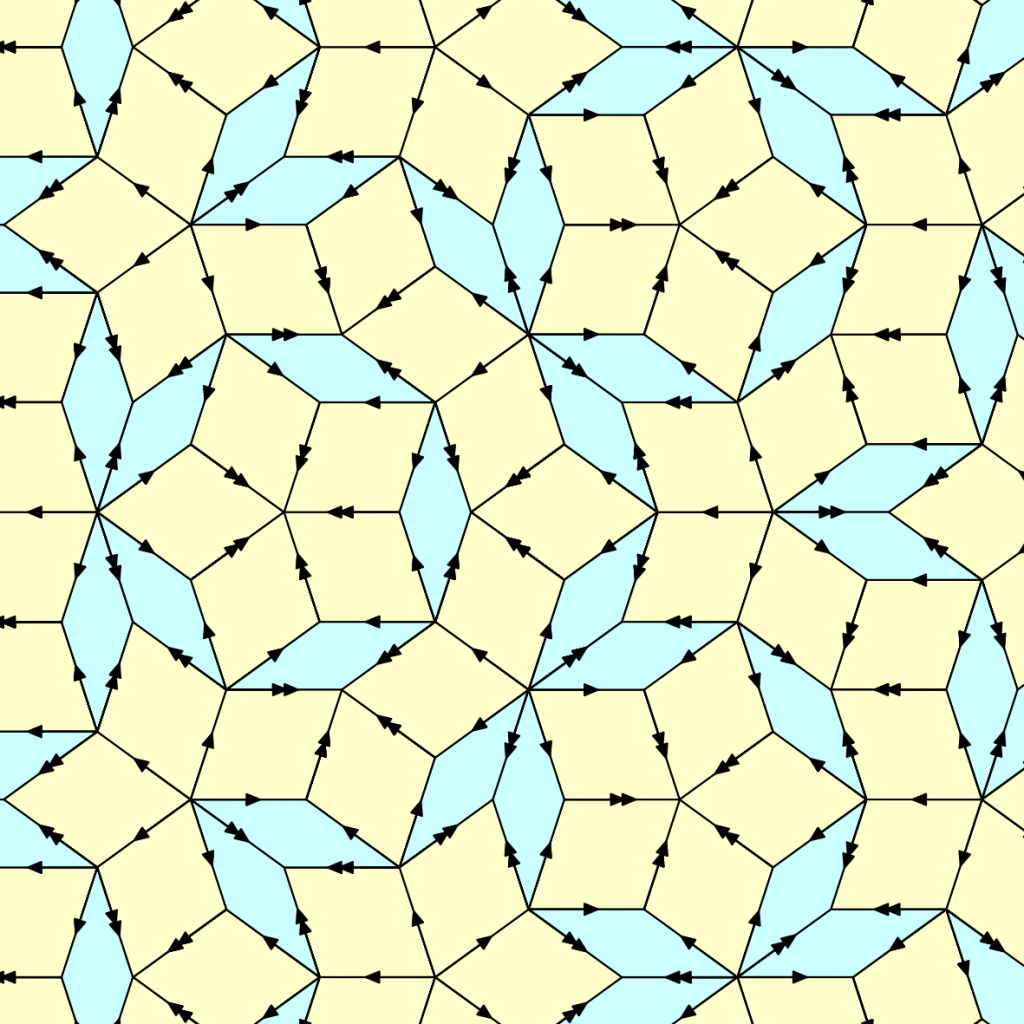Figure 2 : One can tile the entire plane with Penrose tiles respecting the edge rules, and any such tiling must be aperiodic (image courtesy of Durand and Shen).

The Penrose tilings require local rules in the form of edge-matching with decorations. A more geometric version — tiles are polygons, and we want to tile the plane with congruent copies of the tiles with no edge restrictions. This is also possible: see Figure 3.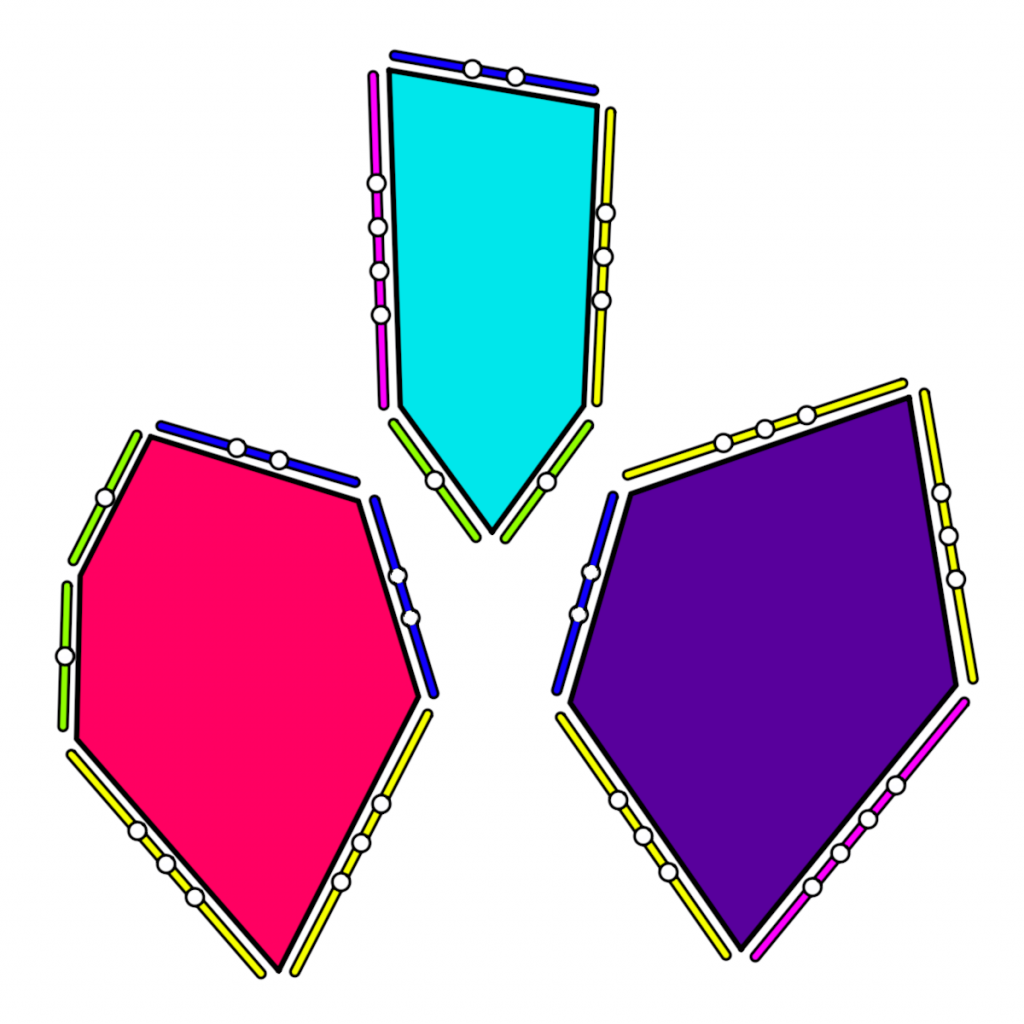Figure 3 : [left] A tiling due to Ammann uses three tiles with matchings forced only by edge lengths. Any tiling of the plane by these tiles is aperiodic. [right] The Ammann tiling is derivable from a Penrose tiling by repartitioning (image courtesy of Durand and Shen). See  for more information.

There are many interesting tile sets: a catalog of them with nice pictures can be found in . However, we concentrate on a more combinatorial version called Wang tiles. In this version all tiles are squares of the same size with colored sides. The translated copies of tiles should form a regular square grid, and the colors of the common edge in two neighbors should be the same.

This version of a tiling problem was introduced by H. Wang in 1960 (while working on the decision problems in the first-order logic, see his account in [3, p. 108]). He conjectured initially that every tile set that admits some tiling must also admit some periodic tiling (invariant under some shift). This conjecture was disproved in 1964 by his student, R. Berger, who constructed a finite tile set that admits some tilings but none periodic: see the thesis  and later published memoir . The construction was quite complicated, and many people tried to simplify it.

The word “simplfy” has different meanings. On may look for the proof from The Book (as Paul Erdős would say), a conceptual proof that would explain why an aperiodic tile sets exist. Here the situation is far from satisfactory: now we know several approaches that seem quite unrelated, and none of them is simple enough to be explained and understood in few minutes. (Who knows: maybe suddenly a completely different and much simpler argument would arise.) But there is a much more quantitative way to measure simplicity: by the size of the aperiodic set of Wang tiles. Here the answer is now known: the minimal aperiodic tile set consists of eleven tiles, as shown by Jeandel and Rao in the paper we review.

THE RESULT OF JEANDEL & RAO. Let us repeat formally the definitions given by Wang, since small details may matter here. [Informal commentaries are in square brackets.] Fix some finite set $C$ whose elements are called colors.

1. A Wang tile is an ordered quadruple of colors; we denote its elements (for a tile $t$) by $t_L$, $t_R$, $t_T$, and $t_B$, where the subscripts connote Left, Right, Top, and Bottom respectively. [Tile $t$ can be visualized as a square whose sides have corresponding colors.]
2. A tileset is a finite set of tiles. [We have infinitely many copies of finitely many tiles.]
3. Given a tileset $\tau$, we define a $\tau$-tiling as a mapping $T\colon\mathbb{Z}^2\to\tau$ that is consistent in the following sense: if $t=T(i,j)$ and $t’=T(i+1,j)$ for some $i$ and $j$, then $t_R=t’_L$; also, if $t=T(i,j)$ and $t’=T(i,j+1)$ for some $i$ and $j$, then $t_T=t’_B$. [Each grid cell with coordinates $(i,j)$ is occupied by a translated copy of tile $T(i,j)$; the right side of the tile has the same color as the left side of its right neighbor, etc.]
4. A tiling $T$ is periodic if it has a period, i.e., there exists a non-zero vector $(u,v)$ such that $T(i,j)=T(i+u,j+v)$ for all $i,j\in\mathbb{Z}$. [Picture remains unchanged with shifted by $(u,v)$.]
5. A tile set $\tau$ is aperiodic if $\tau$-tilings exist but none of them are periodic.

Now we can state the main result of the paper:

THEOREM: [Jeandel-Rao] There exists an aperiodic tile set with $11$ tiles, but no aperiodic tilesets with $10$ or fewer tiles exist.

Here we do not care about the number of colors; in fact the aperiodic set constructed by Jeandel and Rao uses $4$ colors, and they have shown that no tilesets with $3$ or fewer colors (with arbitrary number of tiles) are aperiodic. So their example of an aperiodic tileset is minimal both in the size and in the number of colors.

The reader is probably eager to see this tileset. Here it is, in Figure 4: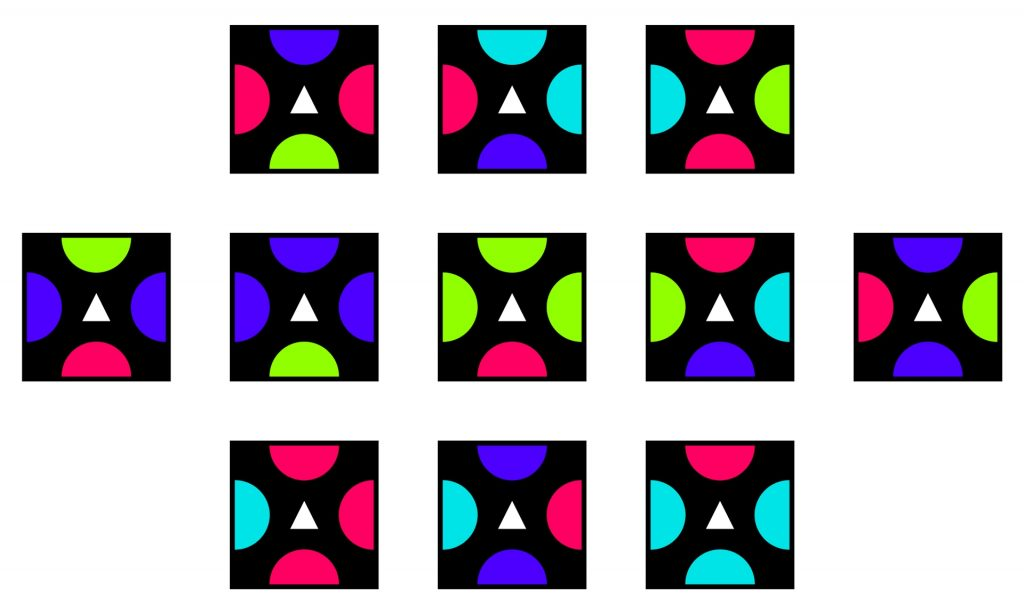Figure 4 : The 11-tile 4-color aperiodic tile set of Jeandel-Rao. Tiles cannot be rotated; only translated.

Frankly speaking, this tileset does not look impressive: no specific structure that could explain why it is aperiodic is visible. To get more ideas about its properties, we may look at an example of tiling (by this tileset), see Figures 5 and 6. Now some structure is visible, but still it looks more like a riddle than an explanation.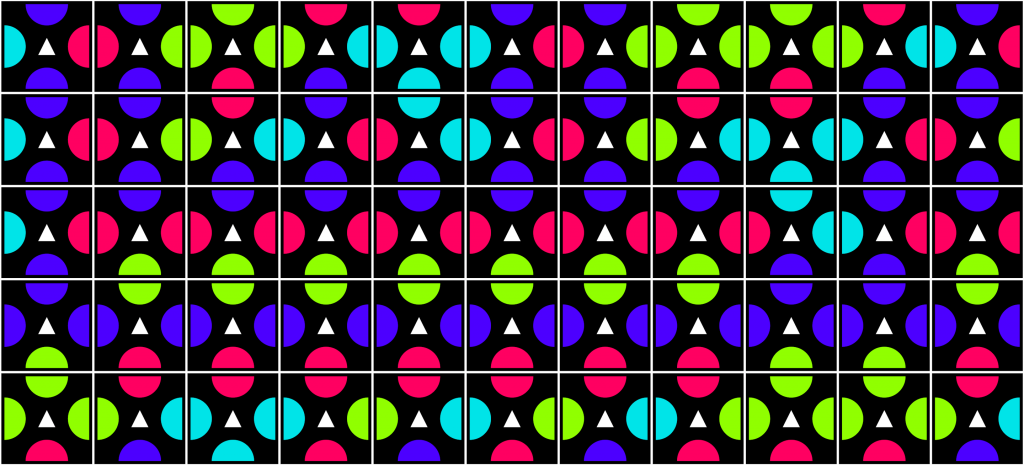Figure 5 : A small portion of a Jeandel-Rao tiling in which all 11 tiles are used at least once.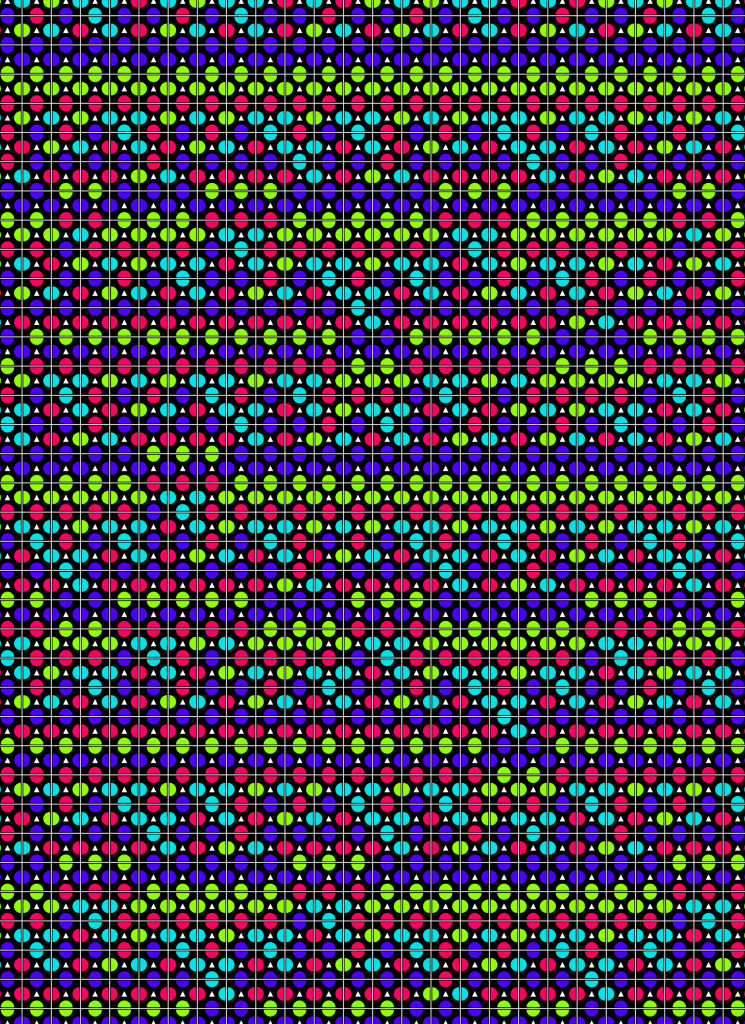Figure 6 : A larger portion of a Jeandel–Rao tiling (derived from an example in their paper) reveals some structure.

HOW THE RESULT WAS OBTAINED. The result looks like a miracle: how one could guess/invent this tileset? How one could check that it is aperiodic? How one could check that all tilesets with $10$ or less tiles are not aperiodic?

The natural approach is to use a computer program. There are finitely many tilesets with fixed number of tiles (up to color renaming). If we had an algorithm that can say whether a tileset is aperiodic, we could (at least theoretically) try this algorithm on all tilesets of sizes $1,2,3,\ldots,10,11$ until we find an aperiodic tileset.

The bad news: such an algorithm does not exist. This was shown in 1960s: the main result in Berger’s paper where an aperiodic tileset was constructed is that the domino problem is undecidable. This means that, given a tileset $\tau$, we cannot algorithmically find out whether $\tau$-tilings exist or not. (In fact, an aperiodic tileset was just a simple byproduct of this main result.) Later Y. Gurevich and I. Koryakov have shown that the “other side” is also undecidable: there is no algorithm that, given a tile set $\tau$, says whether $\tau$ has periodic tilings or not . From their results one can also derive that the combined question whether $\tau$ is an aperiodic tileset or not is also algorithimically undecidable.

There is still good news: both the family of tilesets that have no tilings, and the family of tilesets that have periodic tilings are computably enumerable. This means that there is an algorithm $A$ that, given a tileset $\tau$:

1. If $\tau$ has no tilings, at some point $A$ terminates and outputs “no tilings” ;
2. If $\tau$ admits a periodic tiling, at some point $A$ terminates and outputs “periodic tilings exist” ; and
3. Otherwise (if $\tau$ is an aperiodic tileset) $A$ never terminates.

If we do not care about efficiency, such an algorithm $A$ can be constructed easily. A compactness-type argument (König’s lemma) shows that if $\tau$ has no tilings, then for some $N$ it is impossible to tile correctly the $N\times N$ square, so $A$ may perform an exhaustive search until such a square is found. (Note that for each $N$ there are finitely many tiling candidates.) One can also show that a periodic $\tau$-tiling exists if and only if there exist a $\tau$-tiling of a torus (In fact, tiling of a torus corresponds to a tiling with two independent periods, but one can show that if there is a periodic $\tau$-tiling, a $\tau$-tiling with two independent periods also exists). Again $A$ may perform an exhaustive search until it finds such a tiling for a torus of some size.

Having such an algorithm $A$, we can apply it to all tilesets of size at most $10$, and for each of them $A$ will provide one of the two verdicts (since there are no aperiodic tilesets of that size). So after a finite amount of time we will know that all of these tilesets are not aperiodic (the second part of Jeandel–Rao result). Of course, the problem is that this “finite amount of time” is unreasonably large unless some nontrivial optimizations are made.

Jeandel and Rao used a lot of optimizations. One may find some sufficient conditions for a tileset that guarantee that either there are no tilings, or there exists a periodic tiling, and skip the tilesets that satisfy those conditions. One may try to avoid as much as possible tilesets that are isomorphic to some tileset considered earlier. With all these and many other tricky optimizations it took a significant time (about $4$ days of a computation with about $100$ cores) to exclude all candidates of size at most $10$, except for one (up to isomorphism) problematic case, when the program cannot reach either of two conclusions. Fortunately, this case was quite similar to aperiodic tilesets constructed earlier by J. Kari ($13$ tiles) and K. Čulík ($14$ tiles), so some combination of known tools and computer search allowed Jeandel and Rao to show that tiling does not exist for this last remaining case. Thus, the negative part of Jeandel-Rao result was fully proven. Of course, one could doubt whether all the software and hardware worked correctly during the computation. Jeander and Rao made significant efforts to catch potential errors, e.g., they wrote several programs for the same task and checked that they gave the same answer.

What about the positive part (an aperiodic set of $11$ tiles)? The same technique could be used to exclude most of the candidates — but there are many more tilesets, so much more computational power was used (about one year on several hundred cores). After that, only a few dozens of candidates remained. One of the candidates was analyzed, and the proof of its aperiodicity was discovered. This proof is quite technical; in one sentence it can be described as follows: the horizontal lines can be split into tile blocks of some specific type, and this splitting can be transferred from one horizontal line to another. But the description of these blocks and the splitting is quite technical and requires several tricks.

WHY DO WE CARE? The skeptical reader could say: Well, we know now the exact minimal number of tiles in an aperiodic tileset. So what? Indeed…

1. It would seem that nobody plans to use an aperiodic tileset in some engineering context when the exact size is crucial. (Aperiodic tilesets have a number of applications ranging from quasicrystals in materials science to procedurally-generated textures in computer graphics.)
2. Even if there were an application that depended on the exact number of tiles, the improvement in the number of tiles is not so significant. While the construction of Berger used $20426$ tiles (the other construction of Berger used $104$ tiles, but there was an error that can be corrected and $103$ tiles are used after the correction), this number was made much smaller later: D. Knuth constructed an aperiodic tile set with $92$ tiles (1968), R. Robinson used $56$ tiles (1971), R. Ammann used $16$ tiles (1971), Kari and Čulík used $14$ and $13$ tiles respectively (1996). The latter record held until the Jeandel–Rao work. (For more detailed history of these improvements and the references see Section 1.2 of the Jeandel–Rao paper.) Probably the difference between $11$ and $13$ tiles is not so significant for (hypothetical) applications.
3. The question itself is not very “robust”. For example, we may allow rotations and reflections (not only translations), or replace squares by equilateral triangles. In this way we get a similar problem where aperiodic tilesets (in this new sense) also exist; moreover, there is a reduction that allows one to transform aperiodic tileset of one type into an aperiodic tileset of another type. This transformation does not necessarily preserve the number of tiles, so Jeandel–Rao results do not tell us the minimal size of an aperiodic tileset in these modified settings.

Still there are several reasons why this result is important:

1. There is a sportive aspect (even if one considers it a bit vulgar and does not want to acknowledge it): it is nice to set a world record, but it is even better to set a record that (provably) will never be beaten.
2. All previous constructions of aperiodic tilesets started with some idea of an aperiodicity proof and then this idea was implemented and tiles needed for that implementation were counted. Here the direction is opposite: a given tileset was analyzed and the reason for its aperiodicity was discovered a posteriori — this direction looks much more difficult.
3. The aperiodicity argument used was substantially different from the tools known before (most of the previous proofs were based on self-similarity, with an important exception of Kari and Čulík constructions). This (and the previous) observation gives a hope for a better understanding of the aperiodicity and its underlying reasons.
4. The effect of universality is well known in computation theory and logic: starting from some size, the systems start to exhibit some new (more complicated) type of behavior. Usually we can design an example of a system of this new type (rather large) and prove that for very small system this complicated behavior is impossible — but there is a large gap, a large range of parameters for which we do not know whether such a behavior is possible. The work of Jeandel and Rao shows that (quite unexpectedly) at least in some cases this gap can be filled and we can say exactly what size is necessary for the complicated behavior.

ACKNOWLEDGEMENTS. We thank Thomas Fernique, Peter Gaćs, Emmanuel Jeandel, Nicolas Ollinger, Victor Poupet, Andrei Romashchenko, Pascal Vanier and all the current and former members of the ESCAPE team (Marseille, Montpellier) for many interesting discussions about tilings.

BIBLIOGRAPHY

 T. Sugimoto, Convex polygons for aperiodic tiling, ArXiv:1602.06372.

 D. Frettlöh, E. Harriss, and F. Gähler, Tiling Encyclopedia, https://tilings.math.uni-bielefeld.de/

 H. Wang, Gödel’s and some other examples of problem transmutation, in Perspectives on the History of Mathematical Logic, Birkhauser, 1991, ISBN 978-0-8176-4769-8.

 R. Berger, The undecidability of the domino problem, Ph.D. thesis, Applied Mathematics, Harvard University, Cambridge, MA, July 1964.

 R. Berger, The undecidability of the domino problem, Memoirs of The American Mathematical Society, 1966, ISBN 978-1-4704-0013-2.

 Y. Gurevic, I. Koryakov, Remarks on Berger’s paper on the domino problem, Sib. Math. J., 13, 319–321, 1972 doi.org/10.1007/BF00971620.

.